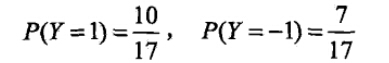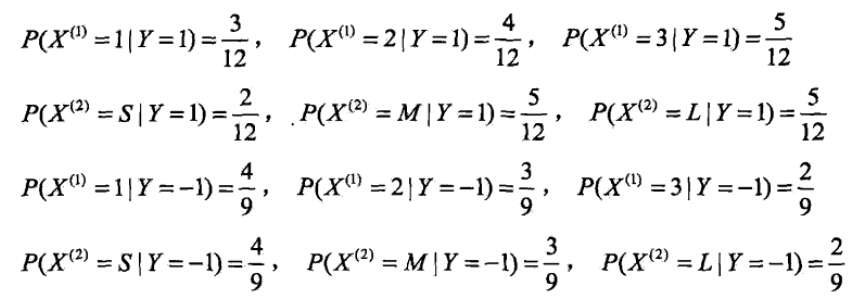# 1. 朴素贝叶斯的理论基础

## 1.1 贝叶斯定理

P(A|B)表示事件B已经发生的前提下，事件A发生的概率，叫做事件B发生下事件A的条件概率。其基本求解公式为： P(A|B)=P(AB)P(B) $P(A|B)=\frac{P(AB)}{P(B)}$

P(B|A)=P(A|B)P(B)P(A) $P(B|A)=\frac{P(A|B)P(B)}{P(A)}$

P(A)=ni=1P(Bi)P(A|Bi) $P(A)=\sum_{i=1}^{n}P(B_{i})P(A|B_{i})$

## 1.2 特征条件独立假设

P(yk|x) $P(y_{k}|x)$怎么求解？答案就是贝叶斯定理：

P(yk|x)=P(x|yk)P(yk)P(x) $P(y_{k}|x)=\frac{P(x|y_{k})P(y_{k})}{P(x)}$

P(yk|x)=P(x|yk)P(yk)kP(x|yk)P(yk) $P(y_{k}|x)=\frac{P(x|y_{k})P(y_{k})}{\sum_{k}P(x|y_{k})P(y_{k})}$ 【公式1】

P(x|yk)=P(x1,x2,...,xn|yk)=ni=1P(xi|yk) $P(x|y_{k})=P(x_{1},x_{2},...,x_{n}|y_{k})=\prod_{i=1}^{n}P(x_{i}|y_{k})$ 【公式2】

P(yk|x)=P(yk)ni=1P(xi|yk)kP(yk)ni=1P(xi|yk) $P(y_{k}|x)=\frac{P(y_{k})\prod_{i=1}^{n}P(x_{i}|y_{k})}{\sum_{k}P(y_{k})\prod_{i=1}^{n}P(x_{i}|y_{k})}$

f(x)=argmaxykP(yk|x)=argmaxykP(yk)ni=1P(xi|yk)kP(yk)ni=1P(xi|yk) $f(x)=argmax_{y_{k}} P(y_{k}|x)=argmax_{y_{k}} \frac{P(y_{k})\prod_{i=1}^{n}P(x_{i}|y_{k})}{\sum_{k}P(y_{k})\prod_{i=1}^{n}P(x_{i}|y_{k})}$

f(x)=argmaxP(yk)ni=1P(xi|yk) $f(x)=argmax P(y_{k})\prod_{i=1}^{n}P(x_{i}|y_{k})$

# 2. 三种常见的模型及编程实现

## 2.1 多项式模型

P(yk)=Nyk+αN+kα $P(y_{k})=\frac{N_{y_{k}}+\alpha}{N+k\alpha}$

N是总的样本个数，k是总的类别个数， Nyk $N_{y_{k}}$是类别为 yk $y_{k}$的样本个数， α $\alpha$是平滑值。

P(xi|yk)=Nyk,xi+αNyk+nα $P(x_{i}|y_{k})=\frac{N_{y_{k},x_{i}}+\alpha}{N_{y_{k}}+n\alpha}$

Nyk $N_{y_{k}}$是类别为 yk $y_{k}$的样本个数，n是特征的维数， Nyk,xi $N_{y_{k},x_{i}}$是类别为 yk $y_{k}$的样本中，第i维特征的值是 xi $x_{i}$的样本个数， α $\alpha$是平滑值。

α=1 $\alpha=1$时，称作Laplace平滑，当 0<α<1 $0<\alpha<1$时，称作Lidstone平滑， α=0 $\alpha=0$时不做平滑。

### 2.1.1 举例• 计算先验概率• 计算各种条件概率• 对于给定的 x=(2,S)T $x=(2,S)^{T}$，计算：### 2.1.2 编程实现（基于Python，Numpy）

"""
Created on 2015/09/06

@author: wepon (http://2hwp.com)

API Reference: http://scikit-learn.org/stable/modules/naive_bayes.html#naive-bayes
"""
import numpy as np

class MultinomialNB(object):
"""
Naive Bayes classifier for multinomial models
The multinomial Naive Bayes classifier is suitable for classification with
discrete features

Parameters
----------
alpha : float, optional (default=1.0)
Setting alpha = 0 for no smoothing
Setting 0 < alpha < 1 is called Lidstone smoothing
Setting alpha = 1 is called Laplace smoothing
fit_prior : boolean
Whether to learn class prior probabilities or not.
If false, a uniform prior will be used.
class_prior : array-like, size (n_classes,)
Prior probabilities of the classes. If specified the priors are not

Attributes
----------
fit(X,y):
X and y are array-like, represent features and labels.
call fit() method to train Naive Bayes classifier.

predict(X):

"""

def __init__(self,alpha=1.0,fit_prior=True,class_prior=None):
self.alpha = alpha
self.fit_prior = fit_prior
self.class_prior = class_prior
self.classes = None
self.conditional_prob = None

def _calculate_feature_prob(self,feature):
values = np.unique(feature)
total_num = float(len(feature))
value_prob = {}
for v in values:
value_prob[v] = (( np.sum(np.equal(feature,v)) + self.alpha ) /( total_num + len(values)*self.alpha))
return value_prob

def fit(self,X,y):
#TODO: check X,y

self.classes = np.unique(y)
#calculate class prior probabilities: P(y=ck)
if self.class_prior == None:
class_num = len(self.classes)
if not self.fit_prior:
self.class_prior = [1.0/class_num for _ in range(class_num)]  #uniform prior
else:
self.class_prior = []
sample_num = float(len(y))
for c in self.classes:
c_num = np.sum(np.equal(y,c))
self.class_prior.append((c_num+self.alpha)/(sample_num+class_num*self.alpha))

#calculate Conditional Probability: P( xj | y=ck )
self.conditional_prob = {}  # like { c0:{ x0:{ value0:0.2, value1:0.8 }, x1:{} }, c1:{...} }
for c in self.classes:
self.conditional_prob[c] = {}
for i in range(len(X)):  #for each feature
feature = X[np.equal(y,c)][:,i]
self.conditional_prob[c][i] = self._calculate_feature_prob(feature)
return self

#given values_prob {value0:0.2,value1:0.1,value3:0.3,.. } and target_value
#return the probability of target_value
def _get_xj_prob(self,values_prob,target_value):
return values_prob[target_value]

#predict a single sample based on (class_prior,conditional_prob)
def _predict_single_sample(self,x):
label = -1
max_posterior_prob = 0

#for each category, calculate its posterior probability: class_prior * conditional_prob
for c_index in range(len(self.classes)):
current_class_prior = self.class_prior[c_index]
current_conditional_prob = 1.0
feature_prob = self.conditional_prob[self.classes[c_index]]
j = 0
for feature_i in feature_prob.keys():
current_conditional_prob *= self._get_xj_prob(feature_prob[feature_i],x[j])
j += 1

#compare posterior probability and update max_posterior_prob, label
if current_class_prior * current_conditional_prob > max_posterior_prob:
max_posterior_prob = current_class_prior * current_conditional_prob
label = self.classes[c_index]
return label

#predict samples (also single sample)
def predict(self,X):
#TODO1:check and raise NoFitError
#ToDO2:check X
if X.ndim == 1:
return self._predict_single_sample(X)
else:
#classify each sample
labels = []
for i in range(X.shape):
label = self._predict_single_sample(X[i])
labels.append(label)
return labels


import numpy as np
X = np.array([
[1,1,1,1,1,2,2,2,2,2,3,3,3,3,3],
[4,5,5,4,4,4,5,5,6,6,6,5,5,6,6]
])
X = X.T
y = np.array([-1,-1,1,1,-1,-1,-1,1,1,1,1,1,1,1,-1])

nb = MultinomialNB(alpha=1.0,fit_prior=True)
nb.fit(X,y)
print nb.predict(np.array([2,4]))#输出-1


## 2.2 高斯模型

### 2.2.1 通过一个例子来说明：

618012
5.9219011
5.5817012
5.9216510
51006
5.51508
5.421307
5.751509

P(身高|性别) x P(体重|性别) x P(脚掌|性别) x P(性别)P(身高=6|男) x P(体重=130|男) x P(脚掌=8|男) x P(男)
= 6.1984 x e-9
P(身高=6|女) x P(体重=130|女) x P(脚掌=8|女) x P(女)
= 5.3778 x e-4

• 总结

P(xi|yk)=12πσ2yk,ie(xiμyk,i)22σ2yk,i $P(x_{i}|y_{k})=\frac{1}{\sqrt{2\pi\sigma_{y_{k},i}^{2}}}e^{-\frac{(x_{i}-\mu_{y_{k},i})^{2}}{2 \sigma_{y_{k},i}^{2}}}$

μyk,i $\mu_{y_{k},i}$表示类别为 yk $y_{k}$的样本中，第i维特征的均值。
σ2yk,i $\sigma_{y_{k},i}^{2}$表示类别为 yk $y_{k}$的样本中，第i维特征的方差。

### 2.2.2 编程实现

#GaussianNB differ from MultinomialNB in these two method:
# _calculate_feature_prob, _get_xj_prob
class GaussianNB(MultinomialNB):
"""
GaussianNB inherit from MultinomialNB,so it has self.alpha
and self.fit() use alpha to calculate class_prior
However,GaussianNB should calculate class_prior without alpha.
Anyway,it make no big different

"""
#calculate mean(mu) and standard deviation(sigma) of the given feature
def _calculate_feature_prob(self,feature):
mu = np.mean(feature)
sigma = np.std(feature)
return (mu,sigma)

#the probability density for the Gaussian distribution
def _prob_gaussian(self,mu,sigma,x):
return ( 1.0/(sigma * np.sqrt(2 * np.pi)) *
np.exp( - (x - mu)**2 / (2 * sigma**2)) )

#given mu and sigma , return Gaussian distribution probability for target_value
def _get_xj_prob(self,mu_sigma,target_value):
return self._prob_gaussian(mu_sigma,mu_sigma,target_value)



## 3 参考文献

03-2301-281万+
03-062327
06-213万+
04-222万+
03-115217
07-15454
06-062482
02-082万+
10-24
09-21870
03-268450
03-14
07-154122
11-261万+
09-17223
06-081064
09-22139